# 1 Introduction

The goal of this package is to encourage the user to try many different clustering algorithms in one package structure, and we provide strategies for creating a unified clustering from these many clustering resutls. We give tools for running many different clusterings and choices of parameters. We also provide visualization to compare many different clusterings and algorithm tools to find common shared clustering patterns. We implement common post-processing steps unrelated to the specific clustering algorithm (e.g. subsampling the data for stability, finding cluster-specific markers via differential expression, etc).

The other main goal of this package is to implement strategies that we have developed in the RSEC algorithm (Resampling-based Sequential Ensemble Clustering) for finding a single robust clustering based on the many clusterings that the user might create by perturbing various parameters of a clustering algorithm. There are several steps to these strategies that we call our standard clustering workflow. The `RSEC` function is our preferred realization of this workflow that depends on subsampling and other ensemble methods to provide robust clusterings, particularly for single-cell sequencing experiments and other large mRNA-Seq experiments.

We also provide a class `ClusterExperiment` that inherits from `SummarizedExperiment` to store the many clusterings and related information, and a class `ClusterFunction` that encodes a clustering routine so that users can create customized clustering routines in a standardized way that can interact with our clustering workflow algorithms.

All of our methods also have a barebones version that allows input of matrices and greater control. This comes at the expense of the user having to manage and keep track of the clusters, input data, transformation of the data, etc. We do not discuss these barebone versions in this tutorial. Instead, we focus on using the `SummarizedExperiment` object as the input and working with the resulting `ClusterExperiment` object. See the help pages of each method for more on how to allow for matrix input.

Although this package was developed with (single-cell) RNA-seq data in mind, its use is not limited to RNA-seq or even to gene expression data. Any dataset characterized by high dimensionality could benefit from the methods implemented here.

## 1.1 The RSEC clustering workflow

The package encodes many common practices that are shared across clustering algorithms, like subsampling the data, computing silhouette width, sequential clustering procedures, and so forth. It also provides novel strategies that we developed as part of the RSEC algorithm (Resampling-based Sequential Ensemble Clustering) .

As mentioned above, RSEC is a specific algorithm for creating a clustering that follows these basic steps:

• Implement many different clusterings using different choices of parameters using the function `clusterMany`. This results in a large collection of clusterings, where each clustering is based on different parameters.
• Find a unifying clustering across these many clusterings using the `makeConsensus` function.
• Determine whether some clusters should be merged together into larger clusters. This involves two steps:
• Find a hierarchical clustering of the clusters found by `makeConsensus` using `makeDendrogram`
• Merge together clusters of this hierarchy based on the percentage of differential expression, using `mergeClusters`.

The basic premise of RSEC is to find small, robust clusters of samples, and then merge them into larger clusters as relevant. We find that many algorithmic methods for choosing the appropriate number of clusters for methods err on the side of too few clusters. However, we find in practice that we tend to prefer to err on finding many clusters and then merging them based on examining the data.

The `RSEC` function is a wrapper around these steps that makes many specific choices in this basic workflow. However, many steps of this workflow are useful for users separately and for this reason, the `clusterExperiment` package generalizes this workflow so that the user can follow this workflow with their own choices. We call this generalization the clustering workflow, as oppose to the specific choices set in `RSEC`.

Users can also run or add their own clusters to this workflow at different stages. Additional functionality for creating a single clustering is also available in the `clusterSingle` function, and the user should see the documentation in the help page of that function.

# 2 Quickstart

In this section we give a quick introduction to the package and the `RSEC` wrapper which creates the clustering. We will also demonstrate how to find features (biomarkers) that go along with the clusters.

## 2.1 The Data

We will make use of a single cell RNA sequencing experiment made available in the `scRNAseq` package.

``````set.seed(14456) ## for reproducibility, just in case
library(scRNAseq)
fluidigm <- ReprocessedFluidigmData()``````

We will use the `fluidigm` dataset (see `help("fluidigm")`). This dataset is stored as a SummarizedExperiment object. We can access the data with `assay` and metadata on the samples with `colData`.

``assay(fluidigm)[1:5,1:10]``
``````##          SRR1275356 SRR1274090 SRR1275251 SRR1275287 SRR1275364 SRR1275269
## A1BG              0          0          0          0          0          0
## A1BG-AS1          0          0          0          0          0          0
## A1CF              0          0          0          0          0          0
## A2M               0          0          0         31          0         46
## A2M-AS1           0          0          0          0          0          0
##          SRR1275263 SRR1275242 SRR1275338 SRR1274117
## A1BG              0          0          0          0
## A1BG-AS1          0          0          0          0
## A1CF              0          0          0          0
## A2M               0          0          0         29
## A2M-AS1           0          0          0        133``````
``colData(fluidigm)[,1:5]``
``````## DataFrame with 130 rows and 5 columns
##               NREADS  NALIGNED    RALIGN TOTAL_DUP    PRIMER
##            <numeric> <numeric> <numeric> <numeric> <numeric>
## SRR1275356  10554900   7555880   71.5862   58.4931 0.0217638
## SRR1274090    196162    182494   93.0323   14.5122 0.0366826
## SRR1275251   8524470   5858130   68.7213   65.0428 0.0351827
## SRR1275287   7229920   5891540   81.4884   49.7609 0.0208685
## SRR1275364   5403640   4482910   82.9609   66.5788 0.0298284
## ...              ...       ...       ...       ...       ...
## SRR1275259   5949930   4181040   70.2705   52.5975 0.0205253
## SRR1275253  10319900   7458710   72.2747   54.9637 0.0205342
## SRR1275285   5300270   4276650   80.6873   41.6394 0.0227383
## SRR1275366   7701320   6373600     82.76   68.9431 0.0266275
## SRR1275261  13425000   9554960   71.1727   62.0001 0.0200522``````
``NCOL(fluidigm) #number of samples``
``##  130``

## 2.2 Filtering and normalization

While there are 130 samples, there are only 65 cells, because each cell is sequenced twice at different sequencing depth. We will limit the analysis to the samples corresponding to high sequencing depth.

``se <- fluidigm[,colData(fluidigm)[,"Coverage_Type"]=="High"]``

We also filter out lowly expressed genes: we retain only those genes with at least 10 reads in at least 10 cells.

``````wh_zero <- which(rowSums(assay(se))==0)
pass_filter <- apply(assay(se), 1, function(x) length(x[x >= 10]) >= 10)
se <- se[pass_filter,]
dim(se)``````
``##  7069   65``

This removed 19186 genes out of 26255. We now have 7069 genes (or features) remaining. Notice that it is important to remove genes with zero counts in all samples (we had 9673 genes which were zero in all samples here). Otherwise, PCA dimensionality reductions and other implementations may have a problem.

Normalization is an important step in any RNA-seq data analysis and many different normalization methods have been proposed in the literature. Comparing normalization methods or finding the best performing normalization in this dataset is outside of the scope of this vignette. Instead, we will use a simple quantile normalization that will at least make our clustering reflect the biology rather than the difference in sequencing depth among the different samples.

``````fq <- round(limma::normalizeQuantiles(assay(se)))
assays(se) <- list(normalized_counts=fq)``````

As one last step, we are going to change the name of the columns “Cluster1” and “Cluster2” that some in the dataset and refer to published clustering results from the paper; we will use the terms “Published1” and “Published2” to better distinguish them in later plots from other clustering we will do.

``````wh<-which(colnames(colData(se)) %in% c("Cluster1","Cluster2"))
colnames(colData(se))[wh]<-c("Published1","Published2")``````

## 2.3 Clustering with RSEC

We will now run `RSEC` to find clusters of the cells using the default settings. We set `isCount=TRUE` to indicate that the data in `se` is count data, so that the log-transform and other count methods should be applied. We also choose the number of cores on which we want to run the operation in parallel via the parameter `ncores`. This is a relatively small number of samples, compared to most single-cell sequencing experiments, so we choose cluster on the top 10 PCAs of the data by setting `reduceMethod="PCA"` and `nReducedDims=10` (the default is 50). Finally, we set the minimum cluster size in our ensemble clustering to be 3 cells (`consensusMinSize=3`). We do this not for biological reasons, but for instructional purposes to allow us to get a larger number of clusters.

Because this procedure is slightly computationally intensive, depending on the user’s machine, we have set this code to not run so that the vignette will compile quickly upon installation. However, it doesn’t take very long (roughly 1-2 minutes) so we recommend users try it themselves. The following code is the example of a common run of RSEC:

``````library(clusterExperiment)
system.time(rsecFluidigm<-RSEC(se, isCount = TRUE,
reduceMethod="PCA", nReducedDims=10,
ncores=1, random.seed=176201))``````

However, to exactly replicate the results here, we will set a large number of parameters to make sure that the vignette is back-compatible in case some defaults change. We will explain these parameters as we go along, but many of these parameters are the default.

``````library(clusterExperiment)
system.time(rsecFluidigm<-RSEC(se,
isCount = TRUE,
k0s = 4:15,
alphas=c(0.1, 0.2, 0.3),
betas = 0.9,
reduceMethod="PCA",
nReducedDims=10,
minSizes=1,
clusterFunction="hierarchical01",
consensusMinSize=3,
consensusProportion=0.7,
dendroNDims=1000,
mergeDEMethod="limma",
mergeCutoff=0.01,
ncores=1,
random.seed=176201))``````

Instead we have saved the results from this call as a data object in the package and will use the following code to load it into the vignette:

``````#don't call this routine if you ran the above code.
#it will overwrite the rsecFluidigm you made
library(clusterExperiment)
data("rsecFluidigm")
``##  '2.1.5.9008'``

### 2.3.1 The output

We can look at the object that was created.

``rsecFluidigm``
``````## class: ClusterExperiment
## dim: 7069 65
## reducedDimNames: PCA
## -----------
## Primary cluster type: mergeClusters
## Primary cluster label: mergeClusters
## Table of clusters (of primary clustering):
##  -1 m01 m02 m03 m04 m05 m06
##  13  15  17  10   4   3   3
## Total number of clusterings: 38
## Dendrogram run on 'makeConsensus' (cluster index: 2)
## -----------
## Workflow progress:
## clusterMany run? Yes
## makeConsensus run? Yes
## makeDendrogram run? Yes
## mergeClusters run? Yes``````

The print out tells us about the clustering(s) that were created (namely 38 clusterings) and which steps of the workflow have been called (all of them have because we used the wrapper `RSEC` that does the whole thing). Recall from our brief description above that RSEC clusters the data many times using different parameters before finding an consensus clustering. All of these intermediate clusterings are saved. Each of these intermediate clusterings used subsampling of the data and sequential clustering of the data to find the clustering, while the different clusterings represent the different parameters that were adjusted.

We can see that `rsecFluidigm` has a built in (S4) class called a `ClusterExperiment` object. This is a class built for this package and explained in the section on ClusterExperiment Objects. In the object `rsecFluidigm` the clusterings are stored along with corresponding information for each clustering. Furthermore, all of the information in the original `SummarizedExperiment` is retained. The print out also tells us information about the “primaryCluster” of `rsecFluidigm`. Each `ClusterExperiment` object has a “primaryCluster”, which is the default cluster that the many functions will use unless specified by the user. We are told that the “primaryCluster” for `rsecFluidigm` is has the label “mergeClusters” – which is the defaul label given to the last cluster of the `RSEC` function because the last call of the `RSEC` function is to `mergeClusters`.

There are many accessor functions that help you get at the information in a `ClusterExperiment` object and some of the most relevant are described in the section on ClusterExperiment Objects. (`ClusterExperiment` objects are S4 objects, and are not lists).

For right now we will only mention the most basic such function that retrieves the actual cluster assignments. The final clustering created by `RSEC` is saved as the `primary` clustering of the object.

``head(primaryCluster(rsecFluidigm),20)``
``##   -1 -1  2  3 -1 -1 -1  1  1  1  2  2 -1  2  4  6 -1  1  4 -1``
``tableClusters(rsecFluidigm)``
``````##
##  -1 m01 m02 m03 m04 m05 m06
##  13  15  17  10   4   3   3``````

The clusters are encoded by consecutive integers. Notice that some of the samples are assigned the value of `-1`. `-1` is the value assigned in this package for samples that are not assigned to any cluster. Why certain samples are not clustered depends on the underlying choices of the clustering routine and we won’t get into here until we learn a bit more about RSEC. Another special value is `-2` discussed in the section on ClusterExperiment objects

This final result of RSEC is the result of running many clusterings and finding the ensembl consensus between them. All of the these intermediate clusterings are saved in `rsecFluidigm` object. They can be accessed by the `clusterMatrix` function, that returns a matrix where the columns are the different clusterings and the rows are samples. We show a subset of this matrix here:

``head(clusterMatrix(rsecFluidigm)[,1:4])``
``````##            mergeClusters makeConsensus k0=4,alpha=0.1 k0=5,alpha=0.1
## SRR1275356            -1            -1             -1             -1
## SRR1275251            -1            -1             -1             -1
## SRR1275287             2             5              3              3
## SRR1275364             3             3             -1             -1
## SRR1275269            -1            -1             -1             -1
## SRR1275263            -1            -1             -1             -1``````

The “mergeClusters” clustering is the final clustering from RSEC and matches the primary clustering that we saw above. The “makeConsensus” clustering is the result of the initial ensembl concensus among all of the many clusterings that were run, while “mergeClusters” is the result of merging smaller clusters together that did not show enough signs of differences between clusters. The remaining clusters are the result of changing the parameters, and a couple of such clusterings a shown in the above printout of the cluster matrix.

The column names are the `clusterLabels` for each clustering and can be accessed (and assigned new values!) via the `clusterLabels` function.

``head(clusterLabels(rsecFluidigm))``
``````##  "mergeClusters"  "makeConsensus"  "k0=4,alpha=0.1" "k0=5,alpha=0.1"
##  "k0=6,alpha=0.1" "k0=7,alpha=0.1"``````

We can see the names of more clusterings, and see that the different parameter values tried in each clustering are saved in the names of the clustering. We can also see the different parameter combinations that went into the consensus clustering by using `getClusterManyParams` (here only 2 different parameters).

``head(getClusterManyParams(rsecFluidigm))``
``````##                clusteringIndex k alpha
## k0=4,alpha=0.1               3 4   0.1
## k0=5,alpha=0.1               4 5   0.1
## k0=6,alpha=0.1               5 6   0.1
## k0=7,alpha=0.1               6 7   0.1
## k0=8,alpha=0.1               7 8   0.1
## k0=9,alpha=0.1               8 9   0.1``````

### 2.3.2 Visualizing the output

`clusterExperiment` also provides many ways to visualize the output of RSEC (or any set of clusterings run in `clusterExperiment`, as we’ll show below).

#### 2.3.2.1 Visualizing many clusterings

The first such useful visualization is a plot of all of the clusterings together using the `plotClusters` command. For this visualization, it is useful to change the amount of space on the left of the plot to allow for the labels of the clusterings, so we will reset the `mar` option in `par`. We also decrease the `axisLine` argument that decides the amount of space between the axis and the labels to give more space to the labels (`axisLine` is passed internally to the `line` option in `axis`).

``````defaultMar<-par("mar")
plotCMar<-c(1.1,8.1,4.1,1.1)
par(mar=plotCMar)
plotClusters(rsecFluidigm,main="Clusters from RSEC", whichClusters="workflow", colData=c("Biological_Condition","Published2"), axisLine=-1)``````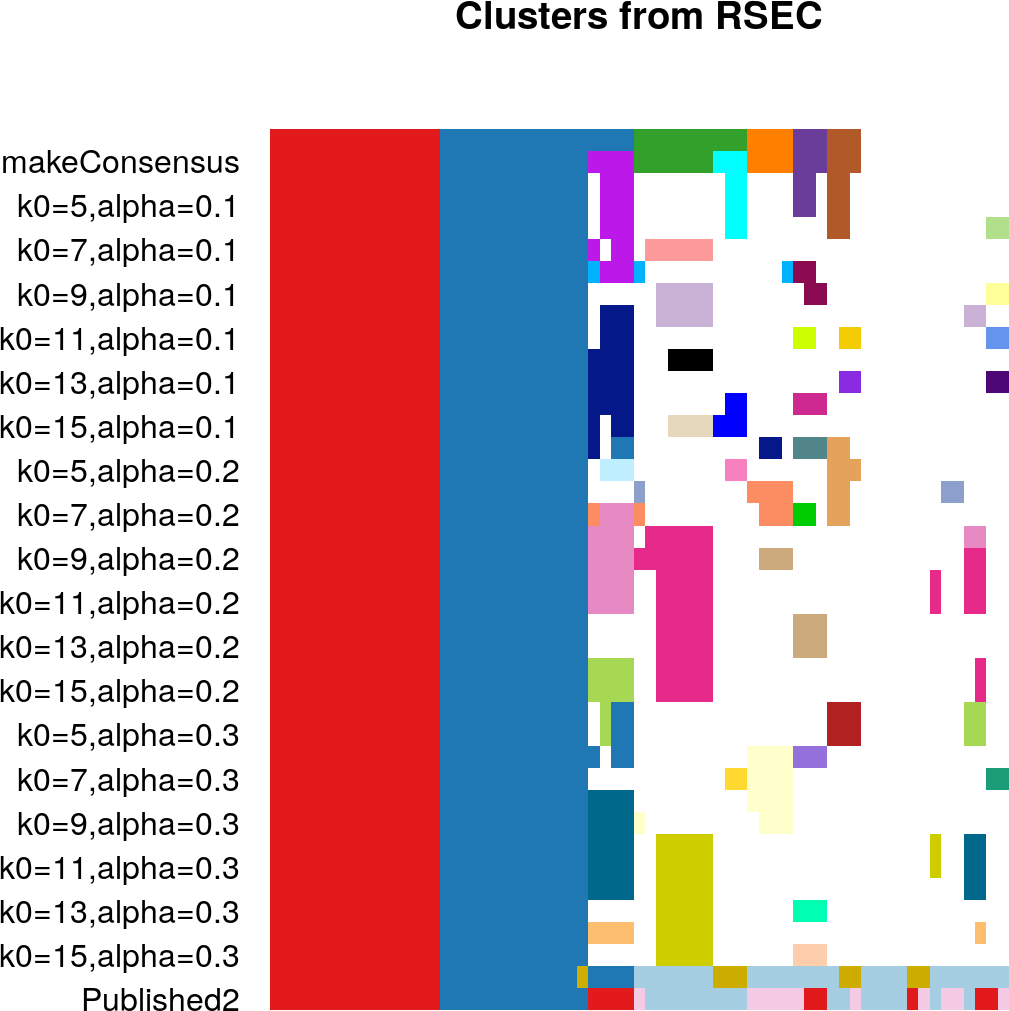This plot shows the samples in the columns, and different clusterings on the rows. Each sample is color coded based on its clustering for that row, where the colors have been chosen to try to match up clusters across different clusterings that show large overlap. Moreover, the samples have been ordered so that each subsequent clustering (starting at the top and going down) will try to order the samples to keep the clusters together, without rearranging the clustering blocks of the previous clustering/row.

We also added a `colData` argument in our call, indicating that we also want to visualize some information about the samples saved in the `colData` slot (inherited from our original `fluidigm` object). We chose the columns “Biological_Condition” and “Published2” from `colData`, which correspond to the original biological condition of the experiment, and the clusters reported in the original paper, respectively. The data from `colData` (when requested) are always shown at the bottom of the plot.

Notice that some samples are white. This indicates that they have the value -1, meaning they were not clustered. In fact, for many clusterings, there is a large amount of white here. This is likely do to the fact that there are only 65 cells here, and the default parameters of RSEC are better suited for a large number of cells, such as seen in more modern single-cell sequencing experiments. The sequential clustering may be problematic for small numbers of cells.

We can use an alternative version of `plotClusters` called `plotClustersWorkflow` that will better emphasize the more final clusterings from the ensemble/concensus step and merging steps (it currently does not allow for showing the `colData` as well, however – only clustering results).

``````par(mar=plotCMar)
plotClustersWorkflow(rsecFluidigm)``````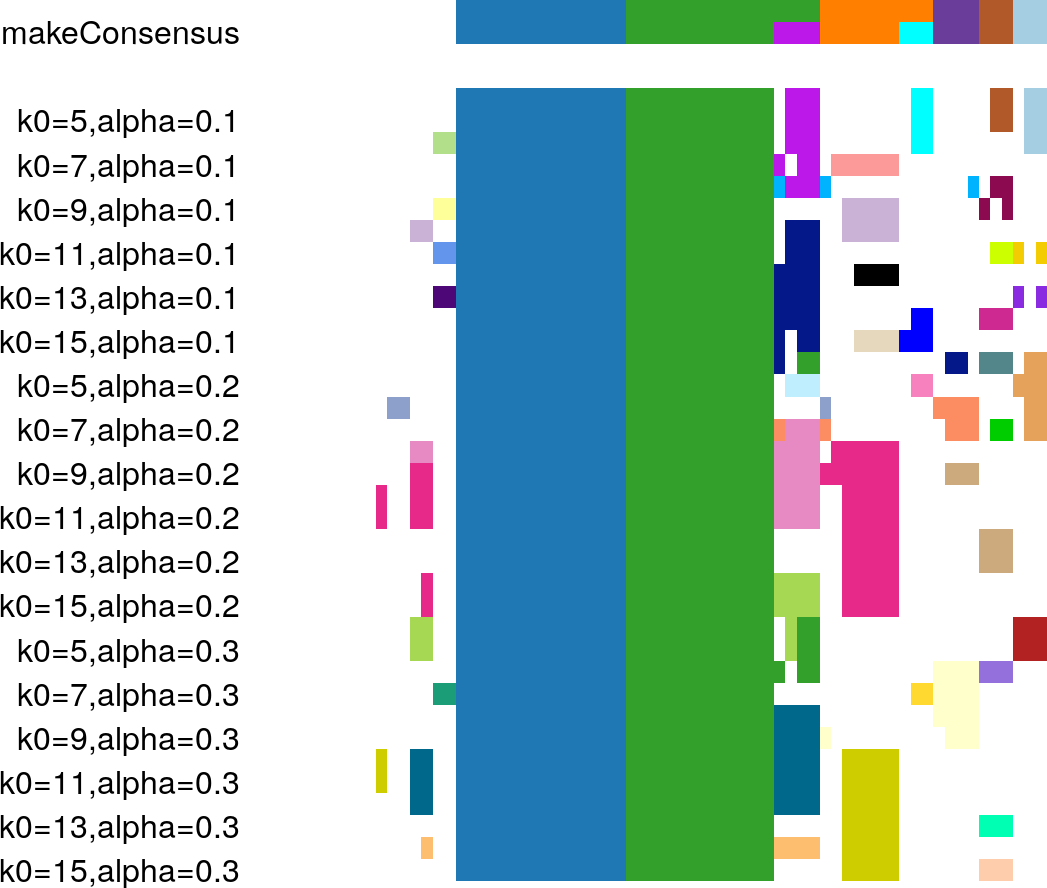#### 2.3.2.2 Barplots & contingency tables

We can examine size distribution of a single clustering with the function `plotBarplot`. By default, the cluster picked will be the primary cluster.

``plotBarplot(rsecFluidigm,main=paste("Distribution of samples of",primaryClusterLabel(rsecFluidigm)))``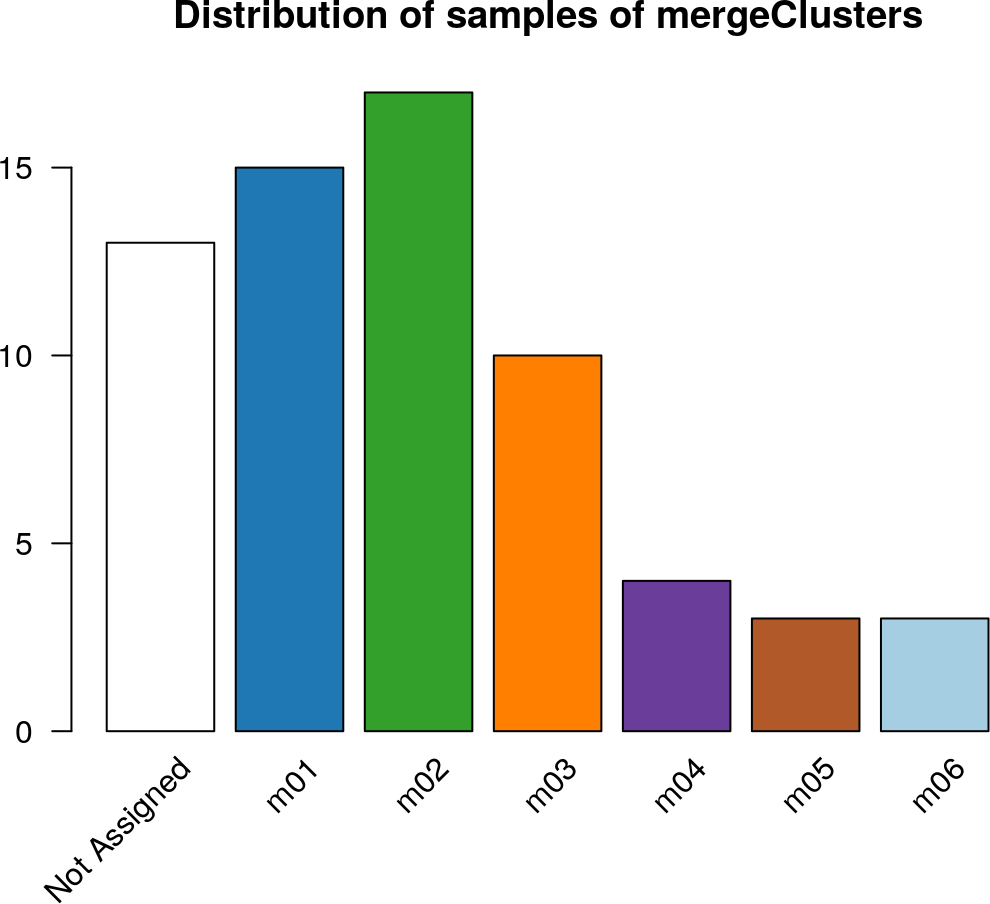We can also pick a particular intermediate clustering, say our intial consensus clustering before merging.

``plotBarplot(rsecFluidigm,whichClusters=c("makeConsensus" ))``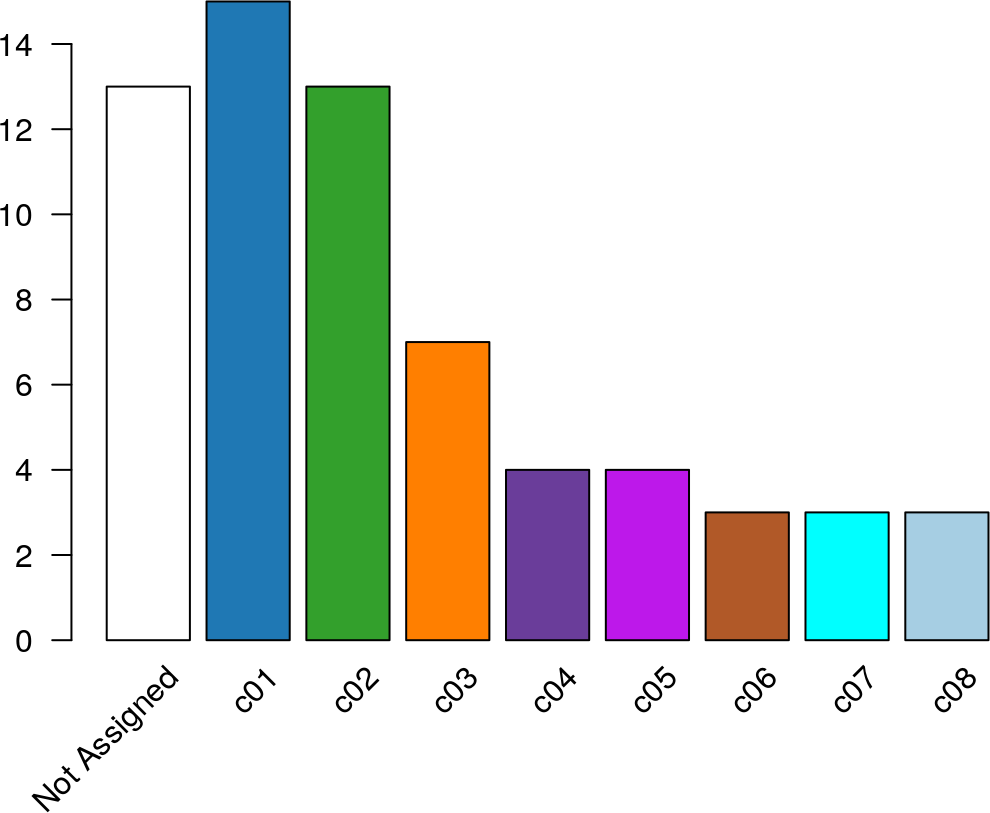We can also compare two specific clusters with a simple barplot using `plotBarplot`. Here we compare the “makeConsensus” and the “mergeClusters” clusterings.

``plotBarplot(rsecFluidigm,whichClusters=c("mergeClusters" ,"makeConsensus"))``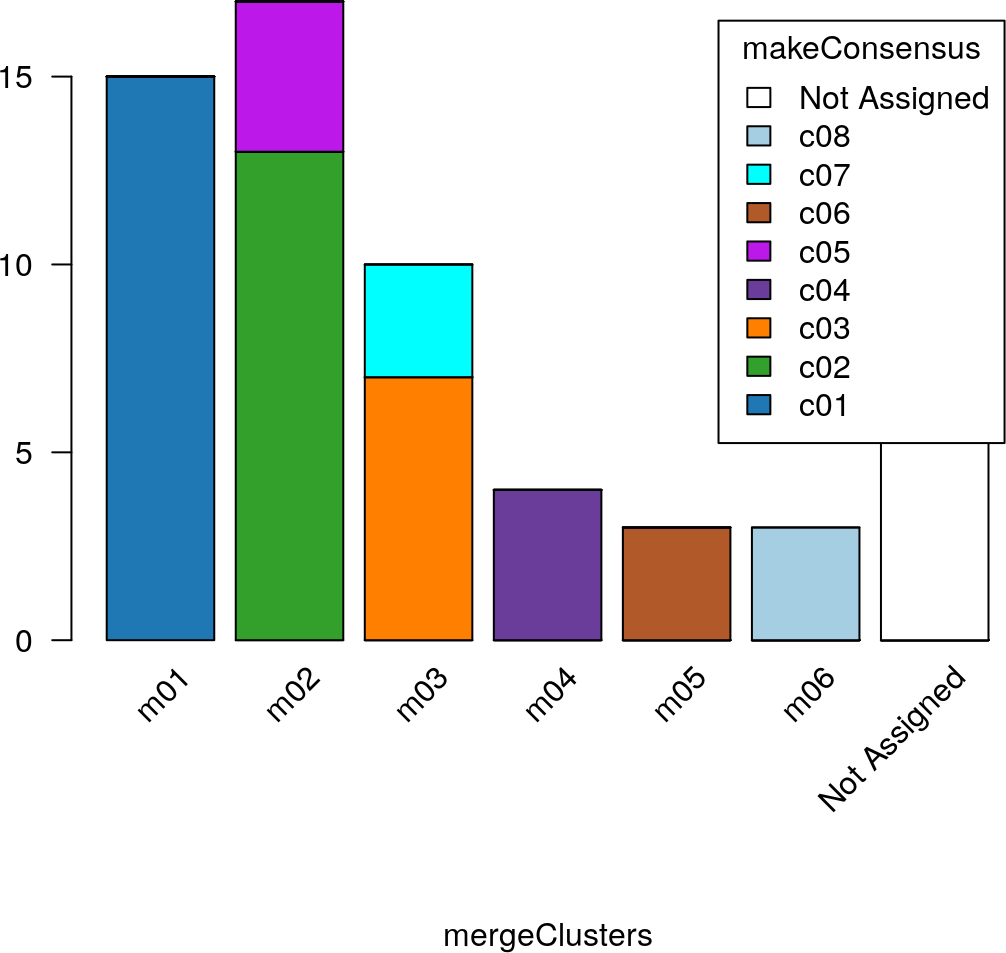Since “makeConsensus” is a partition of “mergeClusters”, there is perfect subsetting within the clusters of “mergeClusters”.

A related plot is to plot a heatmap of the contingency table between two clusterings provided by `plotClustersTable`. This function plots a heatmap of the results of `tableClusters`, optionally converting them to proportions using `prop.table` function based on the parameter `margin`. Here, we’ll set `margin=1`, meaning we will show each row (corresponding to a cluster of the `mergeCluster` clustering), as a proportion – i.e. the grey scale of the heatmap gives (in percentages) how the samples in that row’s cluster are distributed across the clusters of the other clustering, `makeConsensus`

``plotClustersTable(rsecFluidigm,whichClusters=c("mergeClusters" ,"makeConsensus"), margin=1)``